#0
0
01. 云栖社区>
2. paperweekly>
3. 博客>
4. 正文

## 使用PaddleFluid和TensorFlow实现图像分类网络SE_ResNeXt | 文末超大福利

SE 全称 Sequeeze-and-Excitation，SE block 并不是一个完整的网络结构，而是一个子结构，可以嵌到其他分类或检测模型中。SENet block 和 ResNeXt 的结合在 ILSVRC 2017 的分类项目中取得 了第一名的成绩。在 ImageNet 数据集上将 top-5 错误率从原先的最好成绩 2.991% 降低到 2.251%。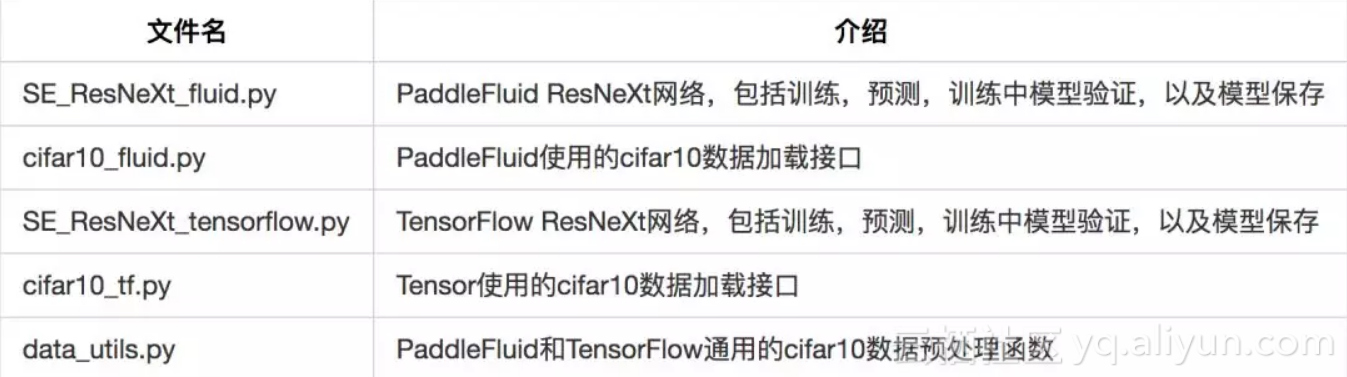``python SE_ResNeXt_fluid.py``

``python SE_ResNeXt_tensorflow.py``

Squeeze-and-Excitation （SE）模块

SE block 由两个非常关键的操作，Squeeze 和 Excitation 构成， 其核心思想是：显式地建模特征通道之间的相互依赖关系，通过自适应学习的方式来获取每个特征通道的重要程度，然后依照这个重要程度去提升有用的特征（有用 feature map 权重增大 ）并抑制对当前任务用处不大的特征（无效或效果小的 feature map 权重减小），达到更好的模型效果。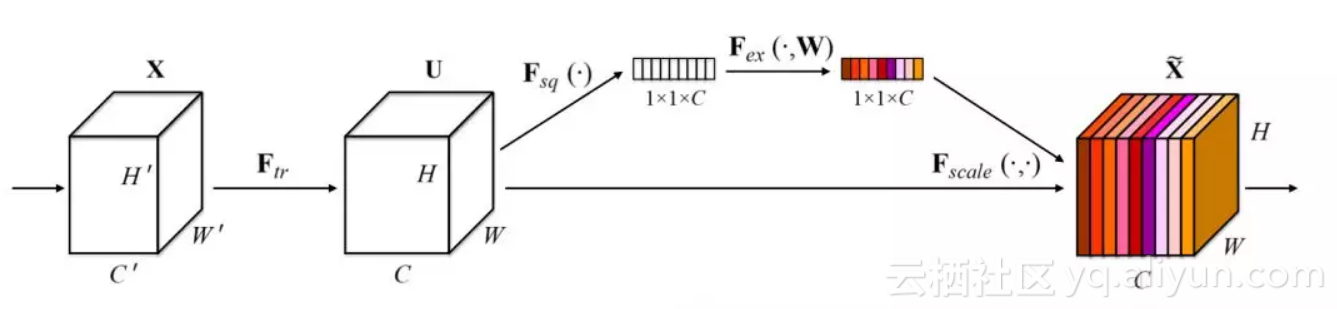Squeeze 操作，图 1 中的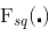Excitation 操作，图 1 中的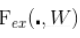Scale 加权操作：

SE模块和Residual Connection叠加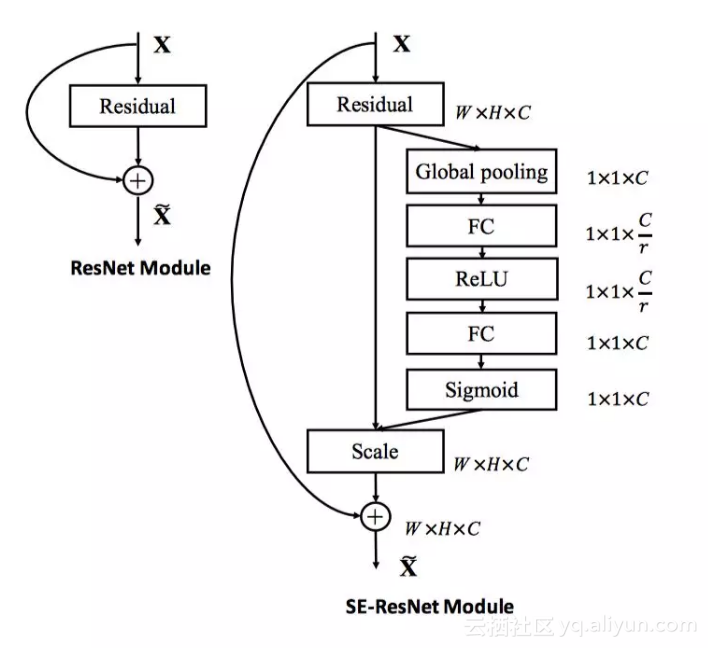SE 模块可以嵌入到含有 Residual Connection 的 ResNet 的模型中，图 2 右是 SE 叠加 Residual Connection 的示意图。论文中建议在 Residual Connection 的跨层连接进行 Addition 操作前，而不是 Addition 操作之后叠加 SE 模块：前对分支上 Residual 的特征进行了特征重标定（recalibration），是由于如果主干上存在 0 ~ 1 的 scale 操作（extraction 步骤），在网络较深时候，反向传播算法进行到网络靠近输入层时，容易出现梯度消散的情况，导致模型难以优化。

ResNeXt 模型结构

Inception 的网络结果经过精心设计，遵循：split-transform-merge 策略。在一个 Inception 模型中：（1）split：输入通过 1×1卷积被切分到几个低维 feature map；（2）transform：split 步骤的输出经过一组（多个）filter size 不同的特定滤波器（filters）映射变换；（3）merge：通过 concatenation 将 transform 步骤的结果融合到一起。

Inception 模型期望通过较低的计算量来近似大的密集的滤波器的表达能力。尽管 Inception 模型的精度很好，但是每个 Inception 模块要量身定制滤波器数量、尺寸，模块在每一阶段都要改变，特别是当 Inception 模块用于新的数据或者任务时如何修改没有特别通用的方法。

ResNeXt 综合了 VGG 和 Inception 各自的优点，提出了一个简单架构：采用 VGG/ResNets 重复相同网络层的策略，以一种简单可扩展的方式延续 split-transform-merge 策略，整个网络的 building block 都是一样的，不用在每个 stage 里对每个 building block 的超参数进行调整，只用一个结构相同的 building block，重复堆叠即可形成整个网络。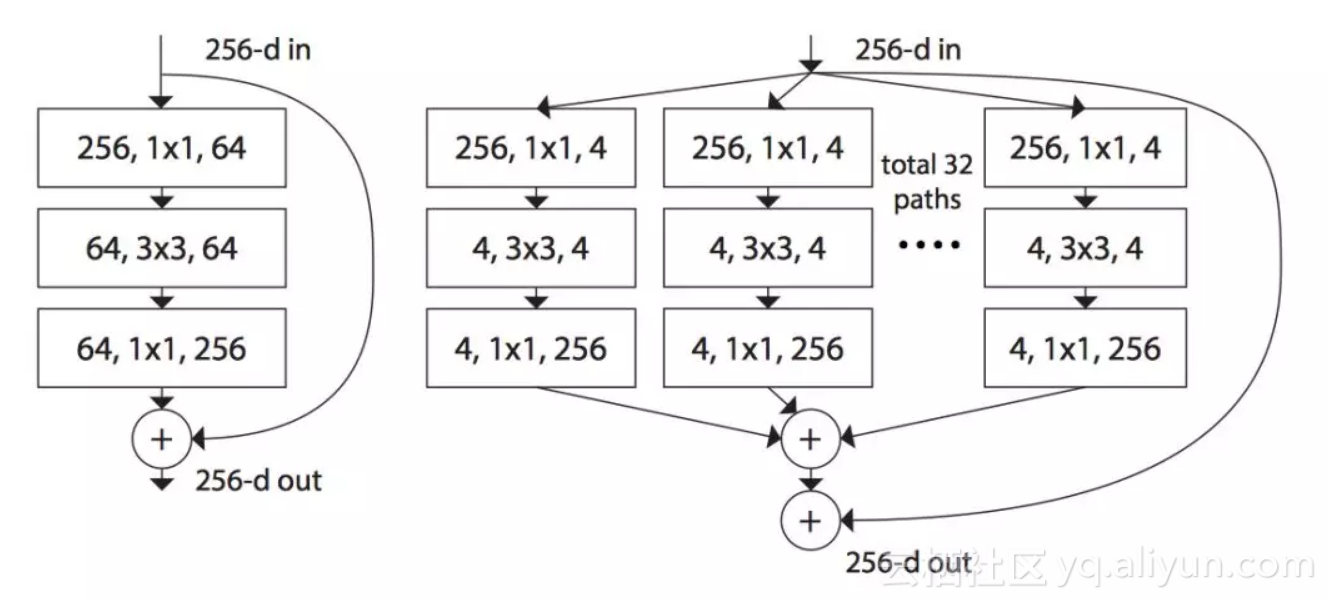CIFAR-10 数据集介绍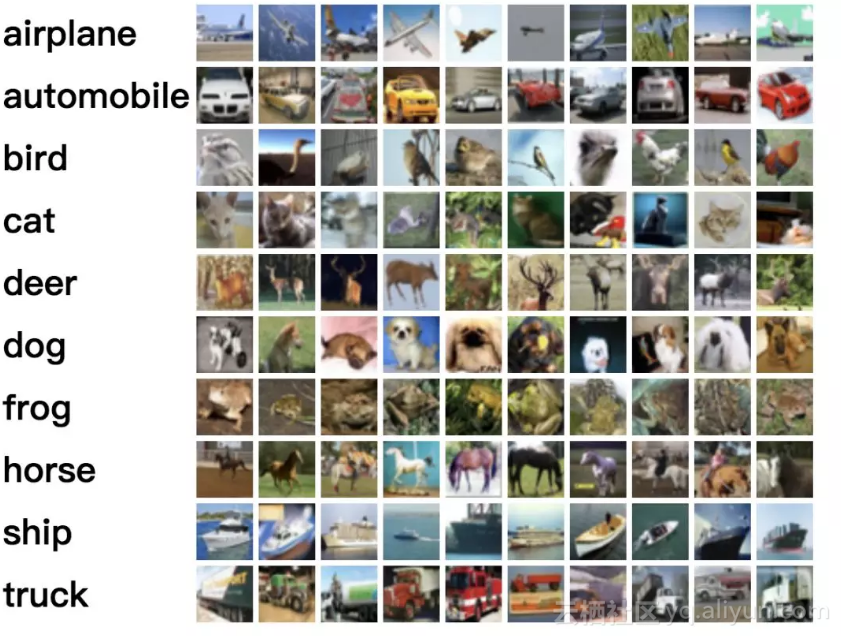cifar10 数据集有 50000 个训练图像和 10000 个测试图像，被划分为 5 个训练块和 1 个测试块，每个块有 10000 个图像。测试块包含从每类随机选择的 1000 个图像。

1. 定义网络的输入层：

``````
``````
`IMG_SHAPE = [3, 32, 32]`
``` ```

``` ```
`images = fluid.layers.data(name="image", shape=IMG_SHAPE, dtype="float32")`
``` ```
`labels = fluid.layers.data(name="label", shape=, dtype="int64")`
``` ```

2. 使用 python 语言编写的 data reader 函数

PaddleFluid 中通过 DataFeeder 接口来为对应的 fluid.data.layersfeed 数据，调用方式如下：

``````
``````
`train_reader = paddle.batch(train_data(), batch_size=conf.batch_size)`
``` ```
`feeder = fluid.DataFeeder(place=place, feed_list=[images, labels])`
``` ```

1. 仅需要用户编写 train_data() 这样一个 python 的 generator。这个函数 是 PaddleFluid 要求提供的 data reader 接口，函数名字不限。

2. 实现这个 data reader 接口时只需要考虑：如何从原始数据文件中读取数据，返回一条以 numpy ndarrary 格式的训练数据

3. 调用 paddle.batch(train_data(), batch_size=conf.batch_size) 接口会将数据首先读入一个 pool 中，进行 shuffle，然后从 pool 中依次取一个一个的 mini-batch。

TensorFlow

1. 定义 placeholder

``````
``````
`IMG_SHAPE = [3, 32, 32]`
``` ```
`LBL_COUNT = 10`
``` ```

``` ```
`images = tf.placeholder(`
``` ```
` tf.float32, shape=[None, IMG_SHAPE, IMG_SHAPE, IMG_SHAPE])`
``` ```
`labels = tf.placeholder(tf.float32, shape=[None, LBL_COUNT])`
``` ```

TensorFlow 中的卷积操作默认使用 channel-last 数据格式（ 也可以在调用卷积的接口中使用 channel-first 的数据格式），images 这个 placeholder 的 shape 和 PaddleFluid 中 images 形状不同。

2. 通过 feeding 字典为placeholder 提供数据

TensorFlow 通过 session 管理运行一个计算图，在 调用 session 的 run 方法时，提供一个 feeding 字典，将 mini-batch 数据 feed 给 placeholder。

``````
``````
`image_train, label_train = train_data()`
``` ```
`image_test, label_test = train_data()`
``` ```
`total_train_sample = len(image_train)`
``` ```

``` ```
`for epoch in range(1, conf.total_epochs + 1):`
``` ```
` for batch_id, start_index in enumerate(`
``` ```
` range(0, total_train_sample, conf.batch_size)):`
``` ```
` end_index = min(start_index + conf.batch_size,`
``` ```
` total_train_sample)`
``` ```
` batch_image = image_train[start_index:end_index]`
``` ```
` batch_label = label_train[start_index:end_index]`
``` ```

``` ```
` train_feed_dict = {`
``` ```
` images: batch_image,`
``` ```
` labels: batch_label,`
``` ```
` ......`
``` ```
` }`
``` ```

``````
``````
`class SE_ResNeXt(object):`
``` ```
` def __init__(self, x, num_block, depth, out_dims, cardinality,`
``` ```
` reduction_ratio, is_training):`
``` ```
` ...`
``` ```

``` ```
` def transform_layer(self, x, stride, depth):`
``` ```
` ...`
``` ```

``` ```
` def split_layer(self, input_x, stride, depth, layer_name, cardinality):`
``` ```
` ...`
``` ```

``` ```
` def transition_layer(self, x, out_dim):`
``` ```
` ...`
``` ```

``` ```
` def squeeze_excitation_layer(self, input_x, out_dim, reduction_ratio):`
``` ```
` ...`
``` ```

``` ```
` def residual_layer(self, input_x, out_dim, layer_num, cardinality, depth,`
``` ```
` reduction_ratio, num_block):`
``` ```
` ...`
``` ```

``` ```
` def build_SEnet(self, input_x):`
``` ```
` ...`
``` ```

SE_ResNeXt_fluid.py  和 SE_ResNeXt_tensorflow.py  SE_ResNeXt 类有着完全相同的成员函数，可以通过对比在两个平台下实现同样功能的代码，了解如何使用经验如何在两个平台之间迁移。

2-D卷积层使用差异

2-D 卷积是图像任务中的一个重要操作，卷积核在 2 个轴向上平移，卷积核的每个元素与被卷积图像对应位置相乘，再求和。卷积核的不断移动会输出一个新的图像，这个图像完全由卷积核在各个位置时的乘积求和的结果组成。图 5 是当输入图像是 4-D Tensor 时（batch size 这里固定是 1，也就是 4-D Tensor 的第一维固定为 1），2-D 卷积的可视化计算过程。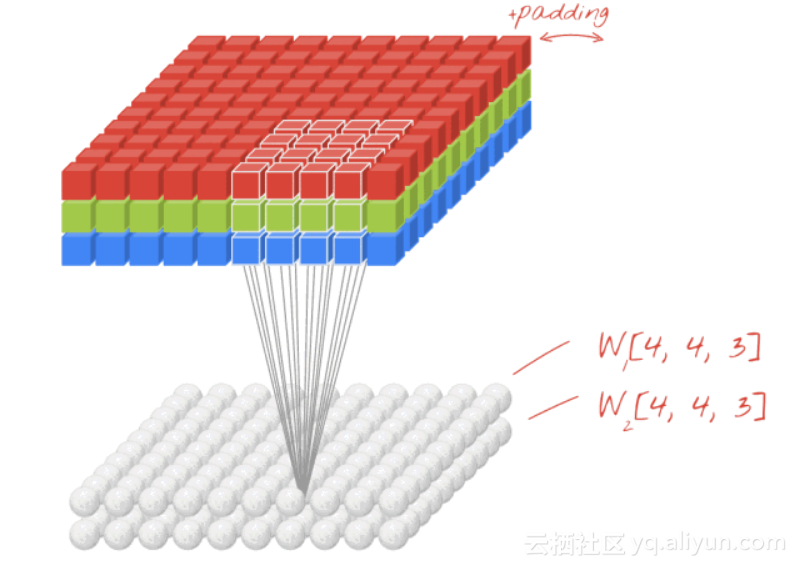``````
``````
`fluid.layers.conv2d(`
``` ```
` input,`
``` ```
` num_filters,`
``` ```
` filter_size,`
``` ```
` stride=1,`
``` ```
` padding=0,`
``` ```
` dilation=1,`
``` ```
` groups=None,`
``` ```
` act=None,`
``` ```
` ...)`
``` ```

2. PaddleFluid 中卷积计算的 padding 属性用户需要自己进行计算，最终输出图像的 height 和 width 由下面公式计算得到：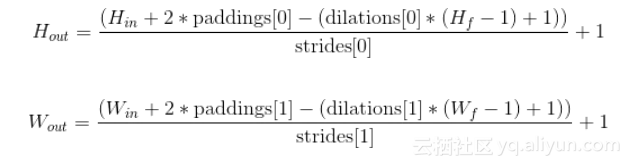padding 属性 可以接受一个含有两个元素的 python list，分别指定对图像 height 方向和width 方向填充。如果 padding 是一个整型数而不是一个 list 使，认为 height width 方向填充相同多个 0。

``````
``````
`tf.layers.conv2d(`
``` ```
` inputs,`
``` ```
` filters,`
``` ```
` kernel_size,`
``` ```
` strides=(1, 1),`
``` ```
` padding='valid',`
``` ```
` data_format='channels_last'`
``` ```
` ...)`
``` ```

1. 在 TensorFlow 卷积默认使用"channel-last"输入数据格式，也就是常说的“NHWC”格式。

2. TensorFlow 中卷积计算的 padding 属性可以指定两种模式：“valid”：不填充；"same"：卷积计算完毕输出图像的宽度和高度与输入图像相同。

L2 正则项作为预防过拟合的手段之一，在神经网络训练中有着重要作用。PaddleFluid 平台和 TensorFlow 中添加 L2 正则的 使用接口略有不同。

``````
``````
`optimizer = fluid.optimizer.Momentum(`
``` ```
` learning_rate=conf.learning_rate,`
``` ```
` momentum=0.9,`
``` ```
` regularization=fluid.regularizer.L2Decay(conf.weight_decay))`
``` ```

TensorFlow

``````
``````
`l2_loss = tf.add_n([tf.nn.l2_loss(var) for var in tf.trainable_variables()])`
``` ```

``` ```
`optimizer = tf.train.MomentumOptimizer(`
``` ```
` learning_rate=learning_rate,`
``` ```
` momentum=conf.momentum,`
``` ```
` use_nesterov=conf.use_nesterov)`
``` ```
`train = optimizer.minimize(cost + l2_loss * conf.weight_decay)`
``` ```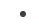定义网络结构；加载训练数据；在一个 for 循环中读取 一个一个 mini-batch 数据，调用网络的前向和反向计算，调用优化过程。

1. 在 PaddleFluid 和 TensorFlow 中如何读取并为网络 feed 图片数据；

2. 如何使用 PaddleFluid 和 TensorFlow 中的 2-d 卷积，2-d pooling，pad 等图像任务中常用计算单元；

3. 如何运行一个完整的训练任务，在训练中对测试集样本进行测试。

+ 关注